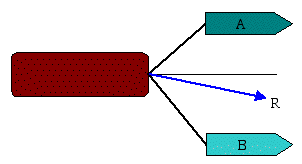Example 1: Two tugboats are towing a cargo ship as shown below. Tugboat A exerts a force of 15,000 N at a 30° angle while tugboat B exerts a force of 20,000 N at a 50° angle. Determine the magnitude and direction of the resultant force acting on the cargo ship.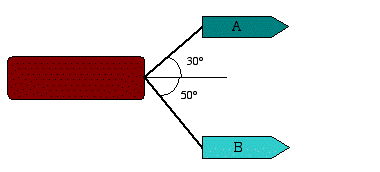Solution: We begin the analysis by drawing the known force vectors. We then construct the force triangle by a head-to-tail connection of the two force components.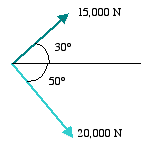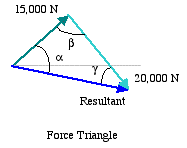Graphical Approach: If the two known sides of the force triangle are drawn to scale, then we can simply measure the length of the resultant vector and multiply it times the scale factor, used for the other two sides, to find its magnitude. To find its direction, we can use a compass to measure its angle from the same reference line.

The accuracy of graphical approach depends on the accuracy in drawing the force triangle and the accuracy in measuring the length and angle of the resultant. Hence, it could be subject to a considerable error.

Trigonometric Approach: An alternative approach is to use the laws of sines and cosines to solve for the resultant. To do this, we need to first determine the angle b in the force triangle. With the help of the force parallelogram shown below, we determine the value of b knowing that the opposite corners of a parallelogram have equal angles.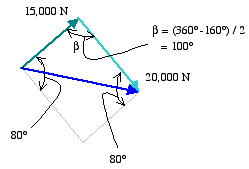With b known, we can use the law of cosines given as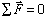to solve for the magnitude of the resultant force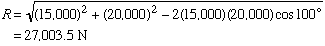We then use the law of sines to solve for angle a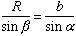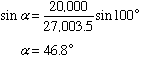Therefore, the direction of the resultant force is 16.8° below the horizontal reference line.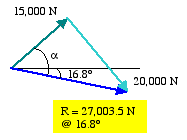Scalar Approach:In this approach, we resolve the force exerted by each tugboat into its x and y components as shown below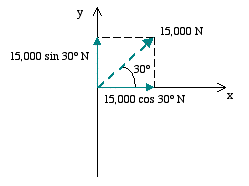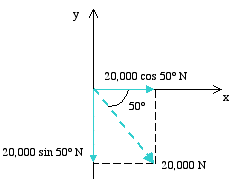We then add the force components in the x direction together, and those in the y direction together to obtain the x and y components of the force resultant, respectively. In doing this, we must pay close attention to the sign convention on individual force components.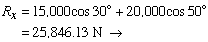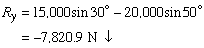With its components known, we can now solve for the magnitude of the force resultant as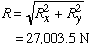The direction of the force resultant, q, is found as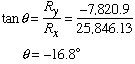Since q is measured positive in the counter clockwise direction from x axis, the force resultant is, therefore, directed below the x axis as shown below.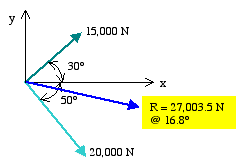We see that the solution found by this approach matches that found by the trigonometric approach. We next examine the vector approach.

Vector Approach: In this approach, each force is represented by its components in the rectangular Cartesian coordinates as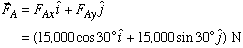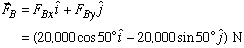We can then solve for the force resultant by adding the two force vectors together.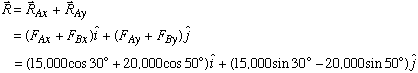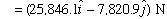The magnitude and direction of the force resultant are then found in the same manner as that described in the scalar approach.

Having found the resultant force vector on the cargo ship, we know its direction of motion.